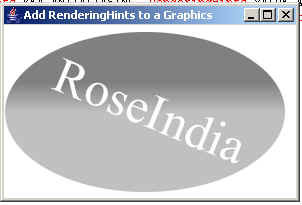Tutorials

# Add RenderingHints to a Graphics

This Java tutorial teaches you about adding the rendering hints to a graphics on the frame. The rendering hints uses the Graphics2D and creates the following image.

This Java tutorial teaches you about adding the rendering hints to a graphics on the frame. The rendering hints uses the Graphics2D and creates the following image.

# Add RenderingHints to a Graphics

This Java tutorial teaches you about adding the rendering hints to a graphics on the frame. The rendering hints uses the Graphics2D and creates the following image.

Description of program:

This program uses the Graphics2D class for rendering hints and draw or construct the given following image.

Description of code:

Graphics2D():
This is the constructor of Graphics2D class that extends the Graphics class to provide

KEY_ANTIALIASING:
This is an antialiasing hint key.

VALUE_ANTIALIAS_ON:
This is a antialiasing hints values and rendered with it.

setRenderingHint():
This method sets the values in Graphics2D object for rendering algorithm.

GradiantPaint(float x1, float y1, Color col1, float x2, float y2, Color col2):
This is the constructor of GradiantPaint class that provides a way for filling the shapes with gradient color pattern. It creates the simple acyclic `GradientPaint` object. It takes the following arguments:

x1: This is the x coordinate of first specified point.
y1: This is the y coordinate of first specified point.
col1: This is the color of first specified point.
x2: This is the x coordinate of second specified point.
y2: This is the y coordinate of second specified point.
col2: This is the color of second specified point.

Ellipse2D():
This is the constructor of an abstract class Ellipse2D that defines bounding rectangle and constructs an ellipse.

transform():
This is the constructor of Transform Class. This is an abstract class that transform
a source tree into a tree result.

Here is the code of program:

 ``` import java.awt.*; import java.awt.event.*; import java.awt.geom.*; public class RanderingHintsGraphics extends Frame{   public static void main(String[] args) {   new RanderingHintsGraphics();   }   public RanderingHintsGraphics(){   setTitle("Add RenderingHints to a Graphics");   setSize(300,200);   setVisible(true);   addWindowListener(new WindowAdapter(){   public void windowClosing(WindowEvent we){   System.exit(0);   }   });   }   public void paint(Graphics g){   Graphics2D d = (Graphics2D)g;   d.setRenderingHint( RenderingHints.KEY_ANTIALIASING, RenderingHints.VALUE_ANTIALIAS_ON);   GradientPaint gpaint = new GradientPaint(   50, 80, Color.gray,50, 110, Color.lightGray);   Ellipse2D el = new Ellipse2D.Double(5, 30, 280, 160);   d.setPaint(gpaint);   d.fill(el);   double rotation = Math.PI/8;   d.transform(AffineTransform.getRotateInstance(rotation));   Font font = new Font("Serif", Font.TRUETYPE_FONT, 50);   d.setFont(font);   d.setPaint(Color.white);   String str = "RoseIndia";   d.drawString(str,80,60);   } }```

`Download this example.`

Output of program: